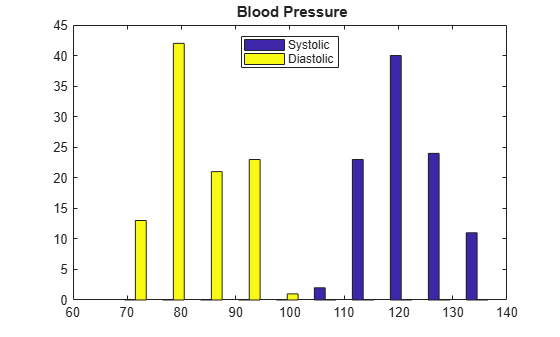# datasetfun

Class: dataset

(Not Recommended) Apply function to dataset array variables

The dataset data type is not recommended. To work with heterogeneous data, use the MATLAB® table data type instead. See MATLAB table documentation for more information.

## Syntax

b = datasetfun(fun,A) [b,c,...] = datasetfun(fun,A) [b,...] = datasetfun(fun,A,...,'UniformOutput',false) [b,...] = datasetfun(fun,A,...,'DatasetOutput',true) [b,...] = datasetfun(fun,A,...,'DataVars',vars) [b,...] = datasetfun(fun,A,...,'ObsNames',obsnames) [b,...] = datasetfun(fun,A,...,'ErrorHandler',efun) 

## Description

b = datasetfun(fun,A) applies the function specified by fun to each variable of the dataset array A, and returns the results in the vector b. The ith element of b is equal to fun applied to the ith dataset variable of A. fun is a function handle to a function that takes one input argument and returns a scalar value. fun must return values of the same class each time it is called, and datasetfun concatenates them into the vector b. The outputs from fun must be one of the following types: numeric, logical, character, structure, or cell.

To apply functions that return results that are nonscalar or of different sizes and types, use the 'UniformOutput' or 'DatasetOutput' parameters described below.

Do not rely on the order in which datasetfun computes the elements of b, which is unspecified.

If fun is bound to more than one built-in function or file, (that is, if it represents a set of overloaded functions), datasetfun follows MATLAB dispatching rules in calling the function. (See Function Precedence Order.)

[b,c,...] = datasetfun(fun,A), where fun is a function handle to a function that returns multiple outputs, returns vectors b, c, ..., each corresponding to one of the output arguments of fun. datasetfun calls fun each time with as many outputs as there are in the call to datasetfun. fun may return output arguments having different classes, but the class of each output must be the same each time fun is called.

[b,...] = datasetfun(fun,A,...,'UniformOutput',false) allows you to specify a function fun that returns values of different sizes or types. datasetfun returns a cell array (or multiple cell arrays), where the ith cell contains the value of fun applied to the ith dataset variable of A. Setting 'UniformOutput' to true is equivalent to the default behavior.

[b,...] = datasetfun(fun,A,...,'DatasetOutput',true) specifies that the output(s) of fun are returned as variables in a dataset array (or multiple dataset arrays). fun must return values with the same number of rows each time it is called, but it may return values of any type. The variables in the output dataset array(s) have the same names as the variables in the input. Setting 'DatasetOutput' to false (the default) specifies that the type of the output(s) from datasetfun is determined by 'UniformOutput'.

[b,...] = datasetfun(fun,A,...,'DataVars',vars) allows you to apply fun only to the dataset variables in A specified by vars. vars is a positive integer, a vector of positive integers, a character vector, a string array, a cell array of character vectors, or a logical vector.

[b,...] = datasetfun(fun,A,...,'ObsNames',obsnames) specifies observation names for the dataset output when 'DatasetOutput' is true.

[b,...] = datasetfun(fun,A,...,'ErrorHandler',efun), where efun is a function handle, specifies the MATLAB function to call if the call to fun fails. The error-handling function is called with the following input arguments:

• A structure with the fields identifier, message, and index, respectively containing the identifier of the error that occurred, the text of the error message, and the linear index into the input array(s) at which the error occurred

• The set of input arguments at which the call to the function failed

The error-handling function should either re-throw an error, or return the same number of outputs as fun. These outputs are then returned as the outputs of datasetfun. If 'UniformOutput' is true, the outputs of the error handler must also be scalars of the same type as the outputs of fun. For example, the following code could be saved in a file as the error-handling function:

function [A,B] = errorFunc(S,varargin) warning(S.identifier,S.message); A = NaN; B = NaN;

If an error-handling function is not specified, the error from the call to fun is rethrown.

## Examples

expand all

Use function handles to compute the mean and plot a histogram of selected variables in a dataset array.

load hospital

Use datasetfun to compute the means of the Weight and BloodPressure variables, and store the results in a dataset array.

stats = datasetfun(@mean,hospital,... 'DataVars',{'Weight','BloodPressure'},... 'UniformOutput',false)
stats=1×2 cell array {} {[122.7800 82.9600]} 

The variable BloodPressure contains two columns: One for the systolic measurement, and one for the diastolic measurement.

Display the mean of the blood pressure variable.

stats{2}
ans = 1×2 122.7800 82.9600 

Plot a histogram of the blood pressure variable.

datasetfun(@hist,hospital,... 'DataVars','BloodPressure',... 'UniformOutput',false); title('{\bf Blood Pressure}') legend('Systolic','Diastolic','Location','N')